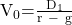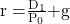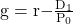Essential Concept 49: Gordon Growth Model | IFT World
101 Concepts for the Level I Exam

# Essential Concept 49: Gordon Growth ModelThe Gordon growth model assumes that:

• Dividends grow at a constant growth rate g.
• Discount rate r is constant and is greater than g.
• Dividends bear and understandable and consistent relationship with profits.

The value of a stock using the Gordon growth model is:If prices are efficient (price equals value), the price is expected to grow at a rate of g, known as the rate of price appreciation. In this case, the stock’s expected rate of return is:If an estimate of the next-period dividend and the stock’s required rate of return are given, then the Gordon growth model can be used to estimate the dividend growth rate implied by the current market price.LIVE WEEKEND CLASSES *For 2024 Exam candidates*
This is default text for notification bar